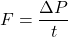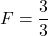Question

During a collision that last 3 seconds, an object has its momentum changed by 3 kgm/s. What was the average Force acting on the object (in Newtons) during this collision

1.F = 1 N

Explanation:

Given,

Change in momentum = 3 kg m/s

time, t = 3 s

Force = ?

We know,

Force is equal to change in momentum per unit time.F = 1 N

Hence, average force acting on the object during collision is equal to 1 N.